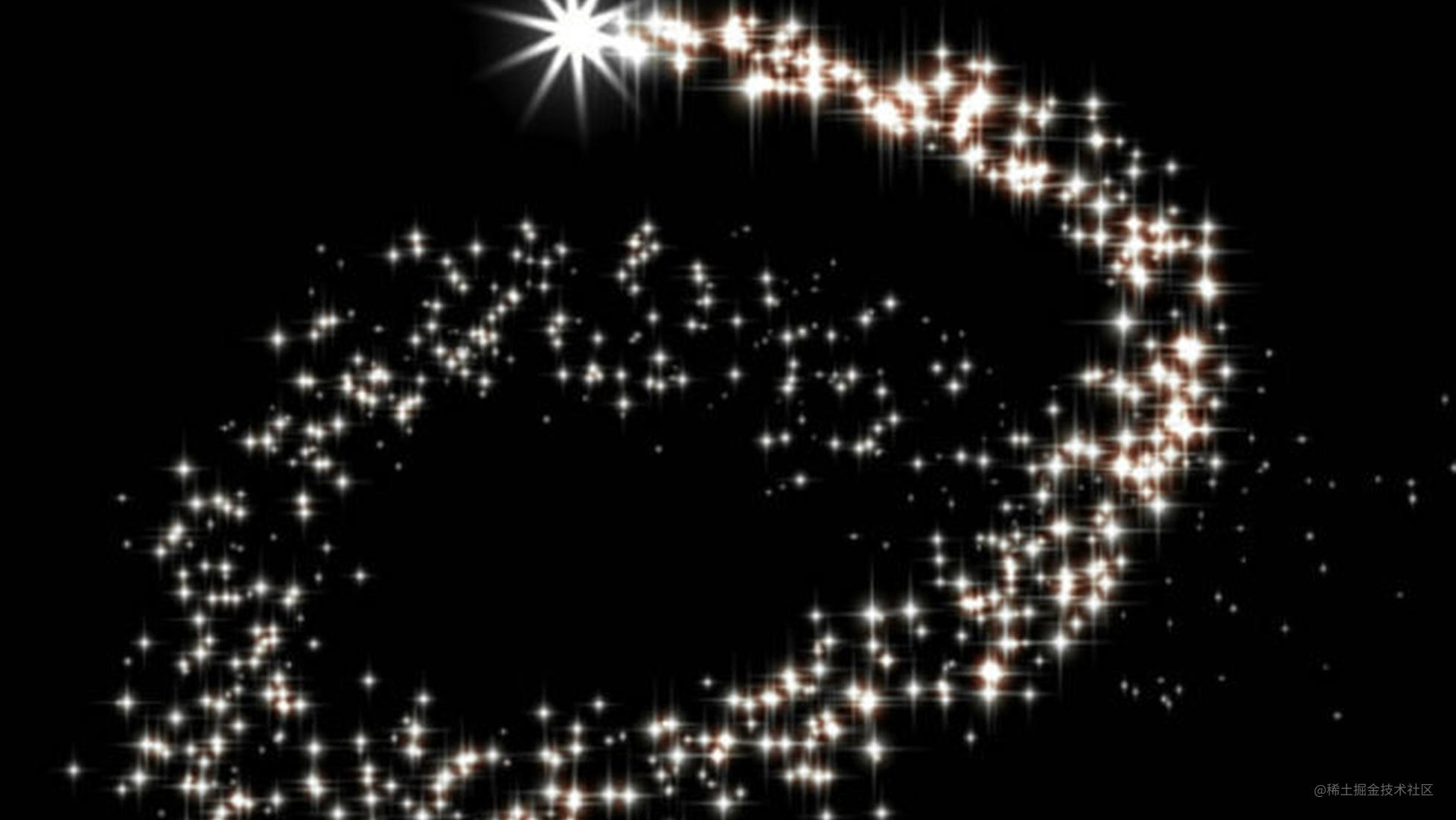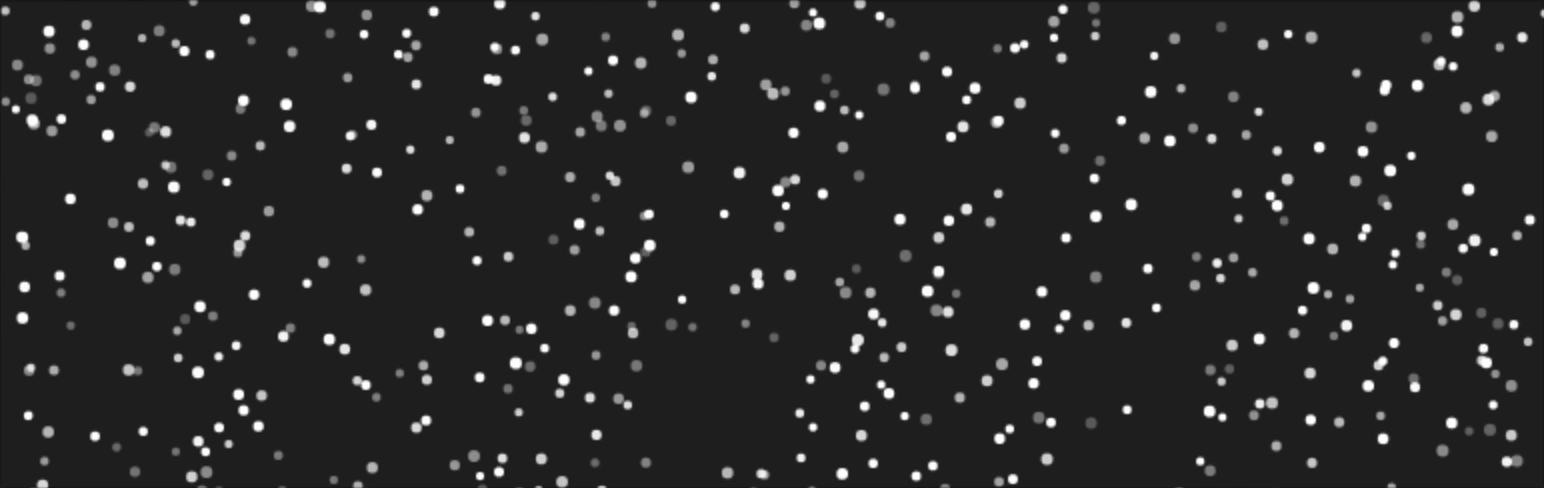# 34 行 JavaScript 代码搞定粒子动画效果

34行JavaScript代码真的能搞定一个粒子动画系统吗？1、速度

2、透明度

3、粒子的尺寸

### 初始化设置

#### HTML

``````<canvas id=c></canvas>

#### CSS

``````canvas {
position: absolute;
top: 0px;
left: 0px;
}

#### JavaScript

``````(()=>{
let \$ = c.getContext("2d"),
w = c.width = window.innerWidth,
h = c.height = window.innerHeight,
//predefines...
})()

``````let pi2 = Math.PI*2,
random = t=>Math.random()*t,
binRandom = t=>Math.random()<t;

``````function draw(){
\$.fillStyle="#222";
\$.fillRect(0,0,w,h);
requestAnimationFrame(draw);
}
draw(); //do not forget to call the function

1、尺寸

2、位置

3、运动速度

4、透明度

``````let arr = new Array(500 /* amount */).fill().map(p=>{
return {
p: {x: random(w), y: random(h) }, //position
v: { //velocity
x: binRandom(0.5)? random(1) : random(-1),
y: binRandom(0.5)? random(1) : random(-1)
},
s: random(1)+2, //size
o: random(1)+.3 //opacity
}
})

1、首先我们创建了一个空的数组。map()方法会遍历数组中的元素并且返回一些指定的值。当然，现在还不能执行这个方法，因为数组是空的。

2、fill()方法则是用于将一个固定值替换数组的元素。

4、粒子的位置position是根据屏幕的宽和高和已经定义好的random方法来计算的。

5、Velocity这个参数主要是用来当粒子撞向可视窗口边缘的时候，使它运动的更加自然而设置的。

6、Size粒子的尺寸也是随机分配的。

7、Opacity，使用透明度是为了使粒子有一个很好的区分。随机分配粒子的透明度的值在.31.3之间。

``````function draw(){
//stuff
arr.forEach(p=>{
\$.fillStyle="rgba(255,255,255,"+p.o+")";
\$.beginPath();
\$.arc(p.p.x, p.p.y, p.s, 0, pi2);
\$.closePath();
\$.fill();
})
}

``````arr.forEach(p=>{
p.p.x += p.v.x;
p.p.y += p.v.y;
if (p.p.x > w || p.p.x < 0) p.v.x *= -1;
if (p.p.y > h || p.p.y < 0) p.v.y *= -1;
//rendering stuff
}

``````(h !== innerHeight || w!==innerWidth) && (w=c.width=innerWidth,h=c.height=innerHeight);